Function Repository Resource:

# BiasedRescale

Bias a value in the unit interval either lower or higher

Contributed by: Jan Mangaldan
 ResourceFunction["BiasedRescale"][a,x] biases x toward lower values if a<1/2 and toward higher values if a>1/2 as x runs from 0 to 1. ResourceFunction["BiasedRescale"][a,x,{min,max}] biases x as x runs from min to max. ResourceFunction["BiasedRescale"][a,x,{min,max},{ymin,ymax}] biases x as x runs from min to max, with the result rescaled to run from ymin to ymax.

## Details and Options

a can be any real number between 0 and 1.
The result of ResourceFunction["BiasedRescale"][a,x,{min,max}] is always between 0 and 1.
ResourceFunction["BiasedRescale"] can take the Method option for the bias function to use.
Possible settings for the Method option are "Schlick" (default) and "Perlin".
With the default option setting Method"Schlick", ResourceFunction["BiasedRescale"] uses Schlick's bias function x/((1/a-2)(1-x)+1).
With Method"Perlin", ResourceFunction["BiasedRescale"] uses Perlin's bias function x-log2(a).
ResourceFunction["BiasedRescale"] is commonly used in computer graphics.

## Examples

### Basic Examples (3)

Evaluate numerically:

 In:=Out=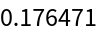Plot over a subset of the reals:

 In:=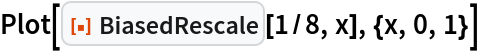Out=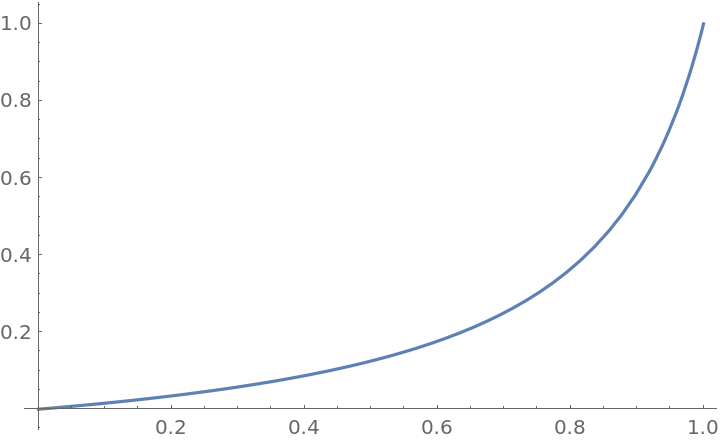Plot different bias functions:

 In:=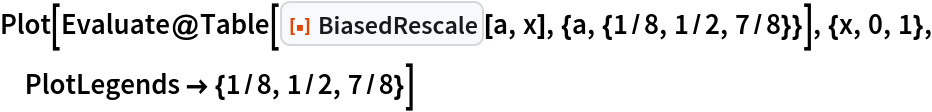Out=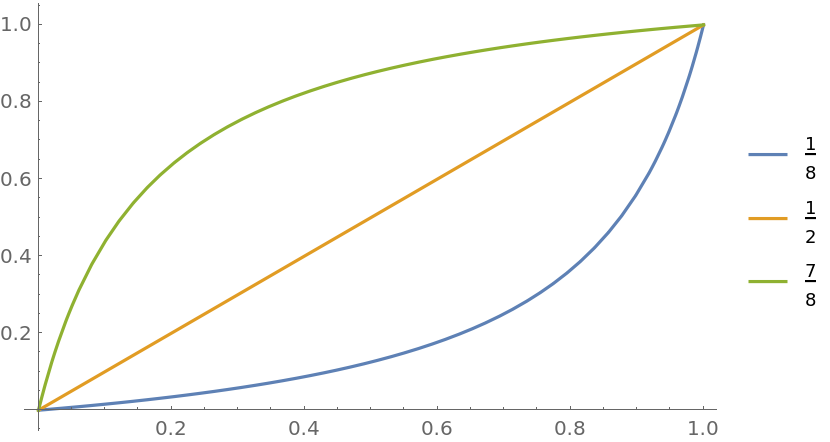### Scope (4)

Evaluate for symbolic x:

 In:=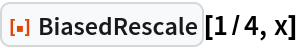Out=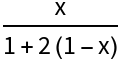BiasedRescale threads over lists in its first and second arguments:

 In:=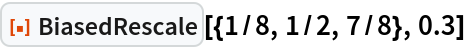Out=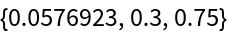In:=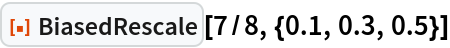Out=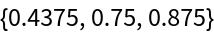Plot different bias functions with a rescaled domain:

 In:=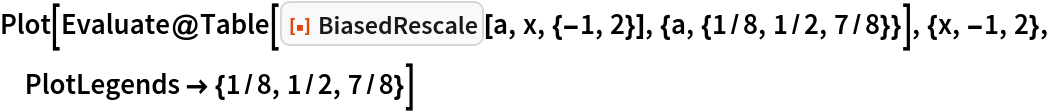Out=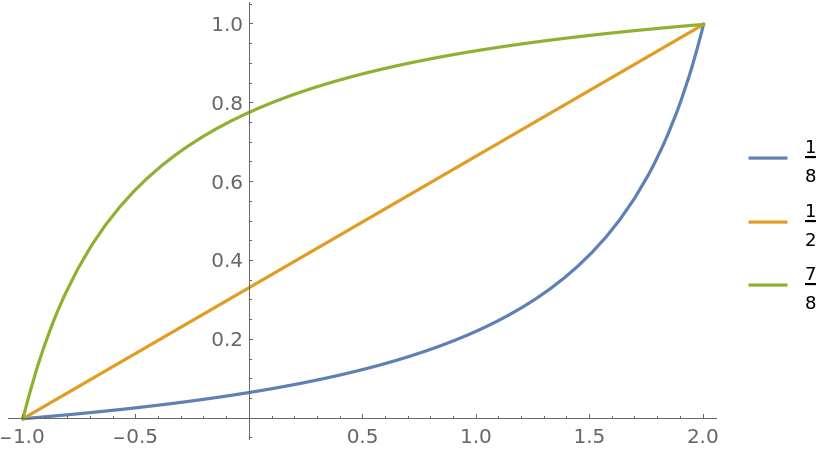Plot different bias functions with a rescaled domain and range:

 In:=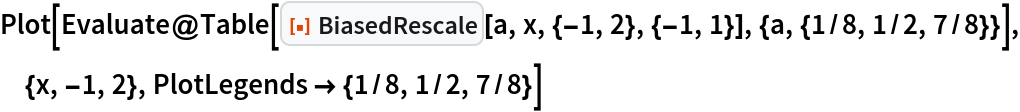Out=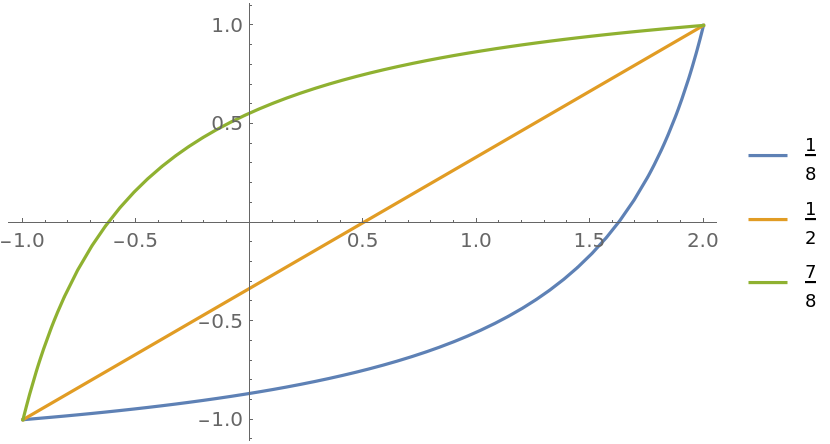### Options (1)

#### Method (1)

Compare the Schlick and Perlin bias functions:

 In:=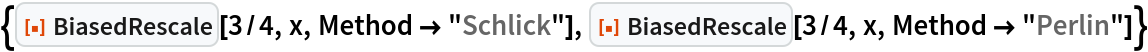Out=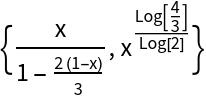In:=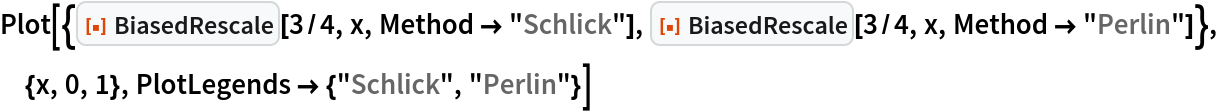Out=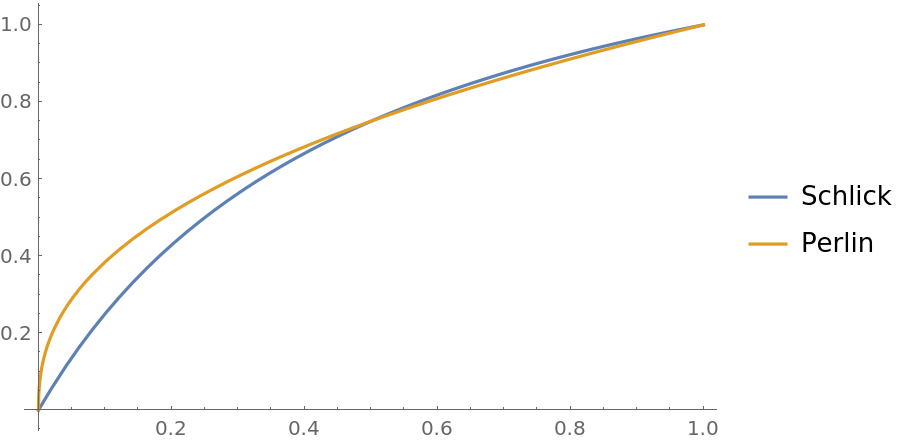### Applications (2)

 In:=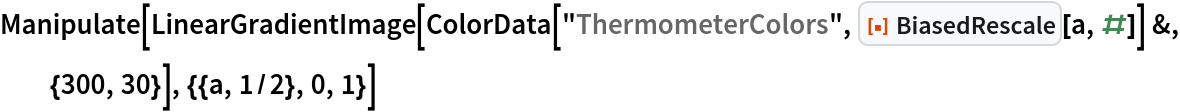Out=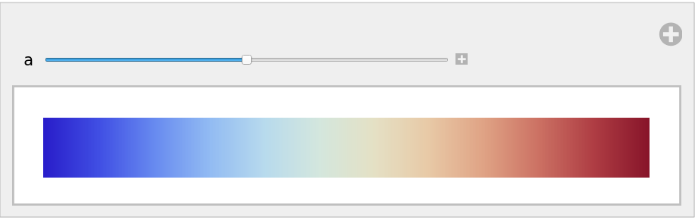Demonstrate the effect of BiasedRescale on easing in/easing out:

 In:=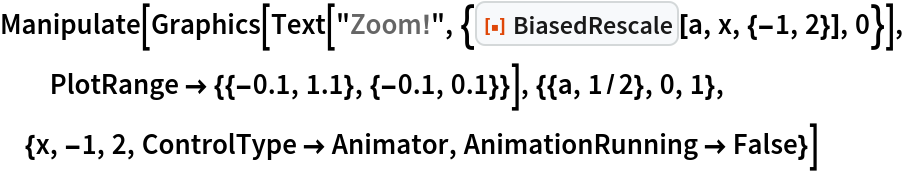Out=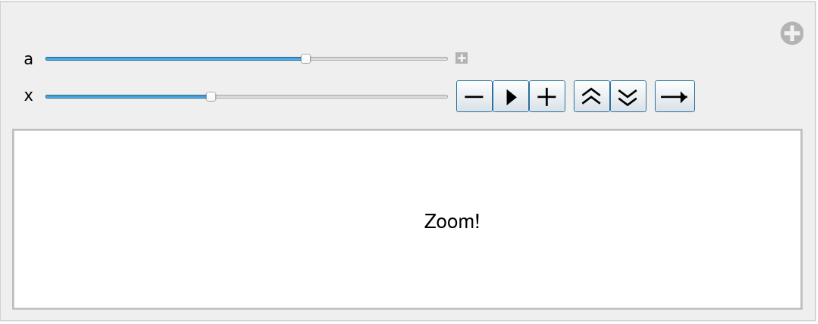### Properties and Relations (1)

BiasedRescale[1/2,x,{min,max}] is equivalent to Rescale[x,{min,max}]:

 In:=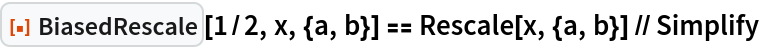Out=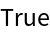### Possible Issues (2)

BiasedRescale is left unevaluated if the first argument is non-numeric:

 In:=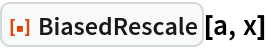Out=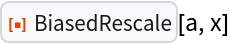BiasedRescale is left unevaluated if the first argument is not a real number between 0 and 1:

 In:=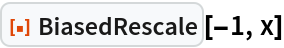Out=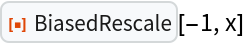### Neat Examples (6)

Use BiasedRescale with the resource function GaussianQuadratureWeights to evaluate a Cauchy principal value integral.

The function to be integrated and the integration limits:

 In:=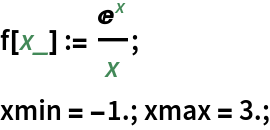Even-order Gaussian quadrature abscissas and weights:

 In:=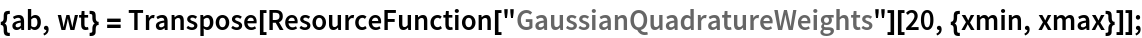The transformation function to be applied to the abscissas and weights:

 In:=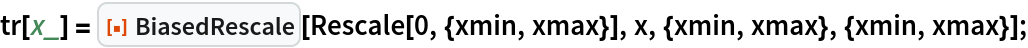Transform the abscissas and weights:

 In:=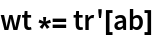Out=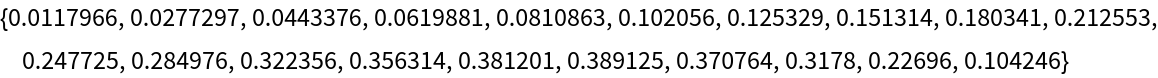In:=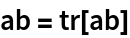Out=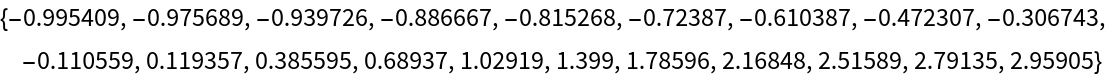Compute the integral:

 In:=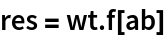Out=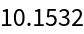Compare with the result of NIntegrate:

 In:=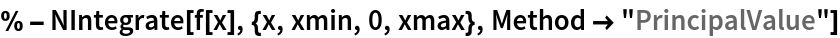Out=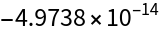## Version History

• 1.0.1 – 16 February 2021
• 1.0.0 – 28 December 2020